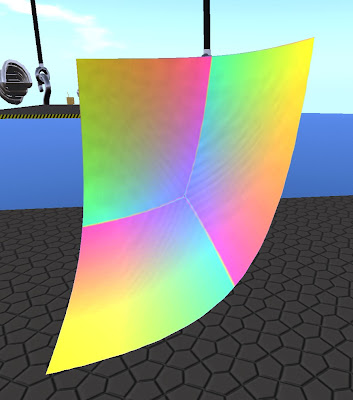## Sunday, March 9, 2008

### Mathematical Sunday with Seifert Surface

Ah, Seifert Surface! Every encounter with that postdoctoral mathematician is enlightening. Take today for example...

Bettina Tizzy: How are things at xyz? Teleport directly to Seifert's new sim, xyz, from here.

Seifert Surface: Just made a sculpty thing which is the graph of the function xyz = 1.It is 24 prims all told. It would be 12 except that plane sculpties are "one sided," so Seifert needed twice as many.

Of course, I had to ask Seifert for a simplified explanation...

Seifert Surface: You have coordinates for points in space, x,y,z. You can ask, what are the points x,y,z so that xyz = 1? That is, you multiply them together and get 1 so, eg: if x, y and z are all 1, it satisfies the equation. 1,1,1 is on my graph, so is 1,-1,-1

Bettina Tizzy: But we are at 500mts, so how do you get 1?

Seifert Surface: Ah, yes, true. The center of this thing is assumed to be 0,0,0 for purposes of the thing, and it goes out to 8 and -8 in each direction... otherwise you wouldn't be able to see any of it. You know like the graph of y = x^2? If you see it on a computer screen, then the origin is not at the bottom left corner of the screen, and the scale is not 1 unit to 1 meter. You can scale and move the graph somewhere else, and it's still the graph of y=x^2. Same thing here. This is just a 3D version of a normal graph.

Bettina Tizzy: What applications can this be given?

Seifert Surface: Well, learning about functions. Unfortunately, it's a real pain to make something like this at present... something that you could use to quickly graph things would be very useful for teaching. You could have an extension to prims, in the same vein as for sculpties. With sculpties, you send a texture to encode the data of the shape. Instead, you could send the mathematical formula for the shape. Then we'd be in business and, of course, animated stuff would work well, too.

Bettina Tizzy: What is this shield item?Seifert Surface: A prototype for the larger piece. I had a smaller range on the graph before, but it looks better with more. That one goes from 0 to 2. The big one is 0 to 8.Bettina Tizzy: Fits better than a glove! More like skin.

Seifert Surface: Should be the exact same function. It fits!